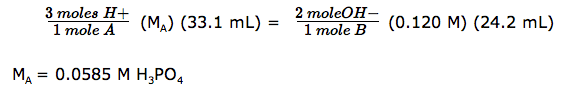# Acids and Bases Test

Fill in the blanks.  Then highlight the paragraph to check your responses.

In a neutral solution, moles of (1)  hydrogen ions   equal the moles of (2)   hydroxide ions  . A pH of 7 indicates that a solution is (3)    neutral   ; a ph less than 7 would mean the solution is (4)    acidic   ; and a pH greater than 7 is a(n) (5)   basic   solution.
Acids have a (6)    sour     taste, react with metals to produce (7)    hydrogen   gas, turn (8)    indicators      different colors according to pH, and are (9)      electrolytes      because their water solutions conduct electricity. On the other hand, bases have a (10)     bitter      taste, feel (11)     slippery     , turn (12)    indicators    different colors according to pH, and are (13)    electrolytes    because their water solutions conduct electricity. Most cleaning products are (14)     basic      , while most foods are (15)     acidic    . Bases turn litmus (16)   blue    , phenolphthalein (17)    hot pink     , and cabbage juice (18)    green     . Acids turn litmus (19)     red    , phenolphthalein (20)     colorless     , and cabbage juice (21)    red (or pink)     .

Choices (may be used more than once)

 acidic basic bitter blue colorless electrolytes green hot pink hydrogen hydrogen ions hydroxide ions Indicators neutral red red (or pink) slippery sour

Match the items on the left to the items on the right. Then click on Answers to check your responses.

 1.   titration A. An acid releases hydrogen ions in a water solution and a base releases hydroxide ions in a water solution. 2.   electrolyte B.  The point in a titration when the indicator changes color. 3.  end point C.  A substance whose water solution conducts an electric current. 4.  salt D.  A laboratory method to determine the concentration of an unknown solution of acid or base using a standard. 5.  Arrhenius definition of an acid and a base E.  A compound formed when the positive ion of a base and the negative ion of an acid during a neutralization reaction.

1. D   2. C  3. B  4. E  5.  A

HI

hydroiodic acid

HNO3

nitric acid

H2SO3

sulfurous acid

H3PO4

phosphoric acid

Name these bases and salts:

KOH

potassium hydroxide

MgSO4

magnesium sulfate

Write formulas for these acids:

hydrofluoric acid

HF

phosphorous acid

H3PO3

Calculate the pH of a 1.4 x 10-2 M NaOH solution.

[H+][OH] = 1.0 x 10-14
[H+][1.4 x 10-2] = 1.0 x 10-14
pH = -log [H+] = -log [7.1 x 10-13]
pH = 12

Calculate the [H+] of a solution with a pH = 3.2.

pH = -log [H+]
3.2 = -log [H+]
[H+] = 6.3 x 10-4 M

Calculate the [OH] of a solution with a [H+] of 9.3 x 10-4 M.

[H+][OH] = 1.0 x 10-14
[9.3 x 10-14][OH] = 1.0 x 10-14
[OH] = 1.0 x 10-11 M

In a titration, 25.0 mL of a 0.20 M NaOH solution is used to neutralize 10.0 mL of HCl.

a. Write the equation for this neutralization reaction.

NaOH + HCl —–> H2O + NaCl

b. Calculate the molarity of the acid.In a titration, 24.2 mL of 0.120 M Mg(OH)2 were required to neutralize 33.1 mL of H3PO4.

a. Write the equation for this neutralization reaction.

2H3PO4 + 3MG(OH)2   ——-> 6H2O +Mg3(PO4)2

b. Calculate the molarity of the acid.What is the word equation for the neutralization of a strong acid and strong base?